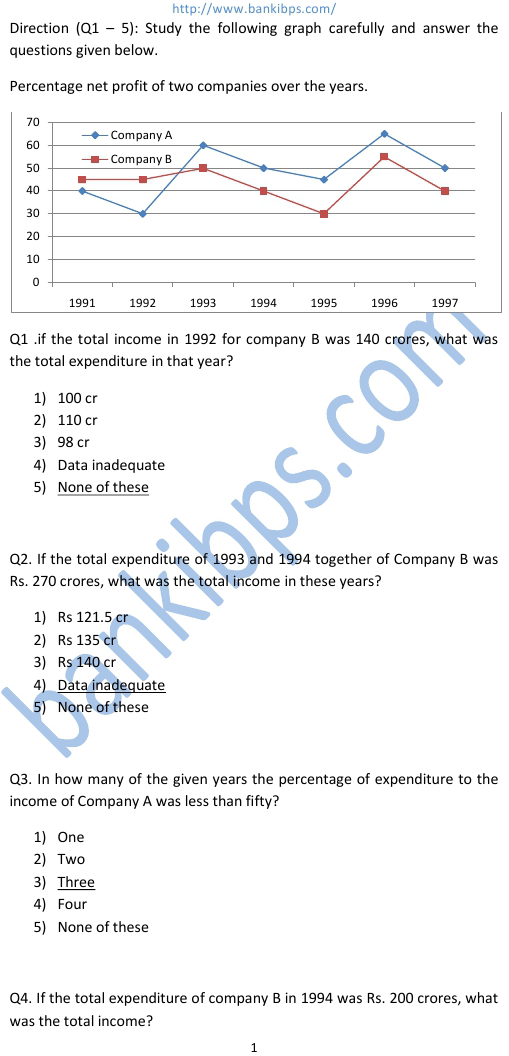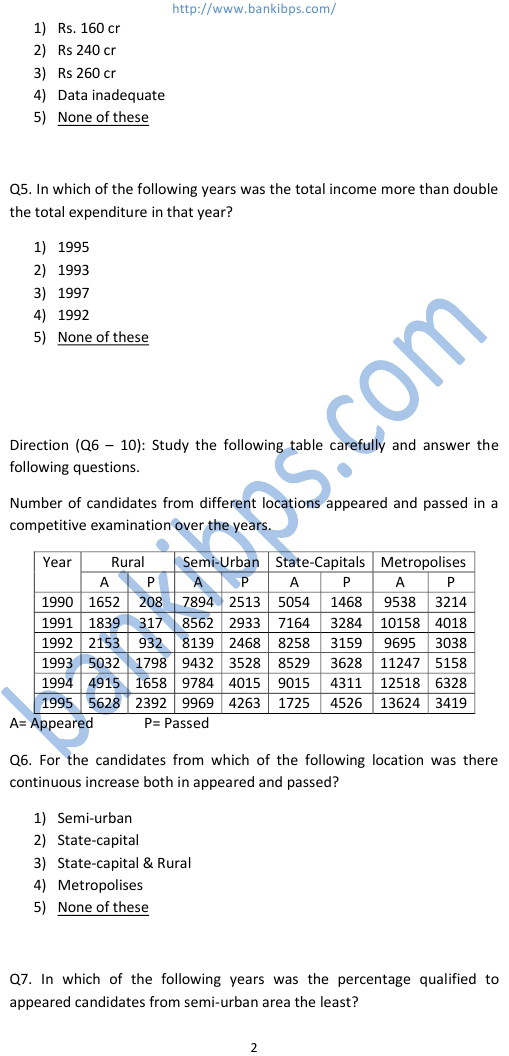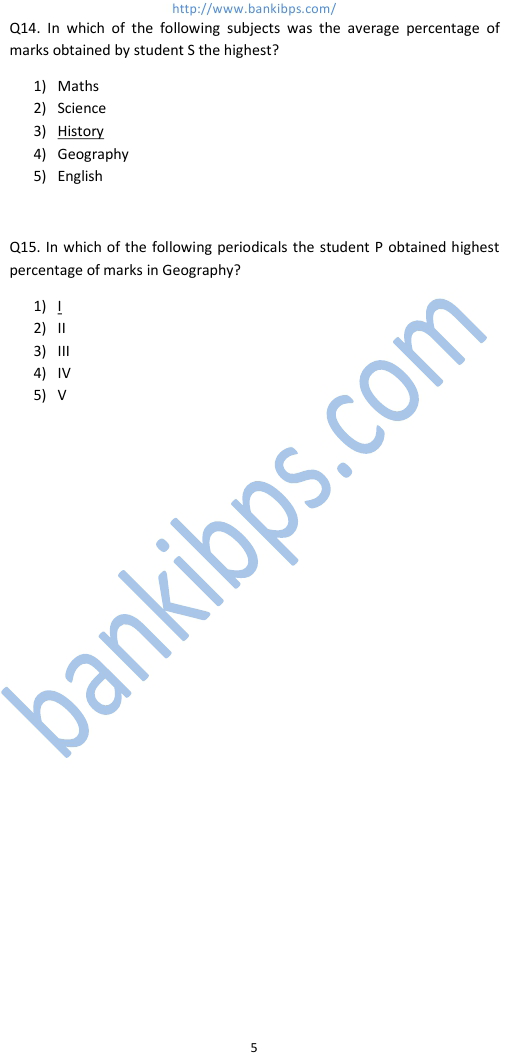# SBI Data Interpretation

Q1 .if the total income in 1992 for company B was 140 crores, what was the total expenditure sbi data interpretation in that year? 1) 100 cr 2) 110 cr 3) 98 cr 4) Data inadequate 5) None of these Q2. If the total expenditure of 1993 and 1994 together of Company B was Rs. 270 crores, what was the total income in these years? 1) Rs 121.5 cr 2) Rs 135 cr 3) Rs 140 cr 4) Data inadequate 5) None of these Q3. In how many of the given years the percentage of expenditure to the income of Company A was less than fifty? 1) One 2) Two 3) Three 4) Four 5) None of these Q4. If the total expenditure of company B in 1994 was Rs. 200 crores, what was the total income?## sbi data interpretation

### sbi data interpretation questions

#### sbi data interpretation and analysis

##### sbi data interpretation paper
###### sbi data interpretation questions with solutions
sbi data interpretation questions. sbi data interpretation and analysis. sbi data interpretation paper. sbi data analysis and interpretation. sbi data interpretation questions with solutions. sbi po data interpretation questions. sbi data interpretation and analysis questions.